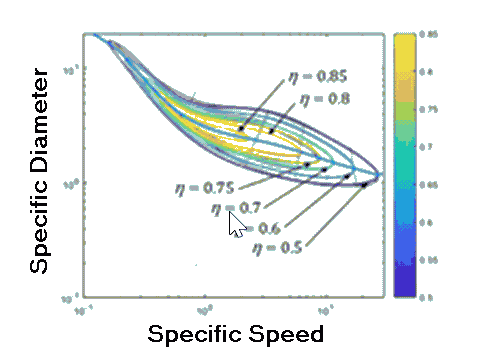Contents - index

Compressor6_CLThe function Compressor6_CL(n_s) uses a curve fit to the Balje curve peak to provide the optimal value of specific diameter for a compressor given the specific speed.  These curves were developed in:

Sondelski, Becky, Mass Optimization of a Supercritical CO2 Brayton Cycle with a Direct Cooled Nuclear Reactor for Space Surface Power, M.S. Thesis, Dept. of Mechanical Engineering, UW-Madison, (2019).

and are based on the Balje curves presented in Balje, O.E., Turbomachinery, New York, Wiley & Sons, (1981).

The calling protocol is:

Compressor6_CL(n_s)

Inputs:

n_s = specific speed (rad) = omega*sqrt(V_dot)/Dh_s^0.75

Output:

d_s = specific diameter (-) = D*Dh_s^0.25/sqrt(V_dot)

Note that the companion function Compressor5_CL provides the efficiency as a function of specific speed and specific diameter.

Example: